# ML Aggarwal Solutions Class 10 Maths Chapter 20 Heights and Distances

1. An electric pole is 10 meter high. if its shadow is 10√3 metres in length, so find out  the elevation of the sun.
Solution:
Let us Assume  AB  as the pole and OB as its shadow.
As we have  given that
AB = 10 m,
OB = 10√3 m
and θ is the angle of elevation of the sun.2. The angle of elevation of the top of a tower from a point on the ground and at a distance of 150 m from its foot is 300 . Find out the height of the tower correct to one place of decimal.
Solution:
Lets Assume,  BC as the tower and A as the point on the ground such that ∠A = 300 and AC = 150 m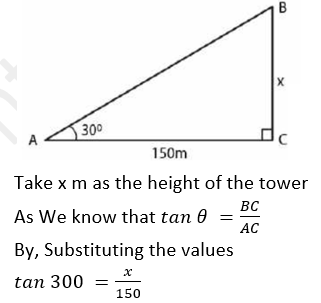3. A ladder is placed against a wall such that it reaches the top of the wall. The foot of the ladder is 1.5 metres away from the wall and the ladder is inclined at an angle of 600 with the ground. Find  out the height of the wall.
Solution:
Lets Assumed, AB as the wall and AC as the ladder whose foot C is 1.5 m away from B Take AB = x m and angle of inclination is 600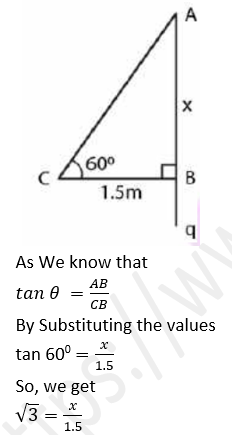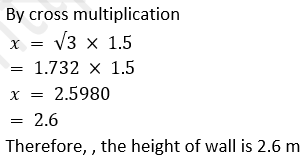4. What is the angle of elevation of the sun when the length of the shadow of a vertical pole is equal to its height.
Solution:
Lets Assume AB as the pole and CB as its shadow
θ is the angle of elevation of the sun
Take AB = x m and BC = x m
We know that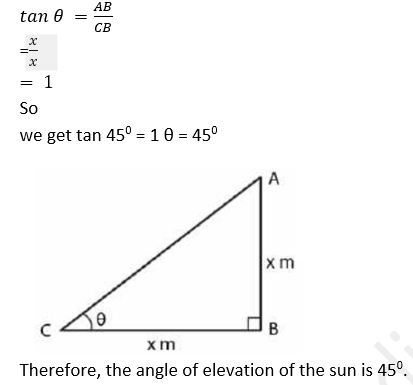5. A river is 60 m wide. A tree of unknown height is on one bank. The angle of elevation of the top of the tree from the point exactly opposite to the foot of the tree on the other bank is 300 . Find out the height of the tree.
Solution:
Lets Assume  AB as the tree and BC as the width of the river
C is the point which is exactly opposite to B on the other bank and 300 is the angle of elevation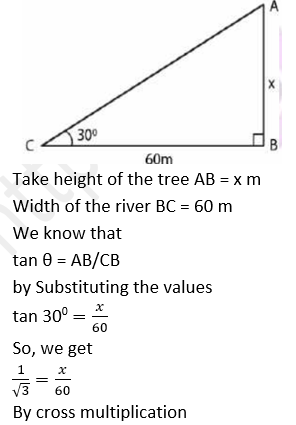6. From a point P on level ground, the angle of elevation of the top of a tower is 30° . If the tower is 100 m high, how much far is P from the foot of the tower?
Solution:

Lets Assume  AB as the tower and P is at a distance of x m from B which is the foot of the tower. Height of the tower = 100 m
Angle of elevation = 30°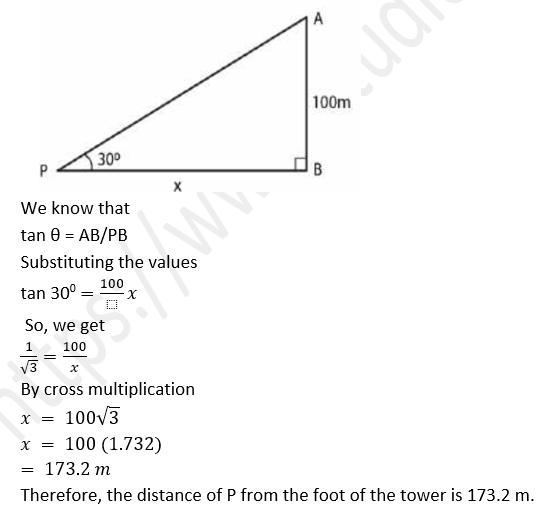7. From the top of a cliff 92 m high, the angle of depression of a buoy is 20° . Find out to the nearest meter, the distance of the buoy from the foot of the cliff.
Solution:
Lets Assume, AB as the cliff whose height is 92 m C is buoy making depression angle of 20°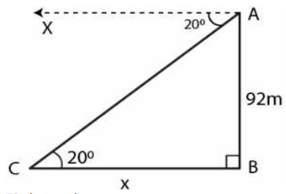As We know that
∠ACB = 20°
Take CB = x m
In a right angle triangle ABC
cot θ =BC/AB
Substituting the values
cot 20°  = x/92
By cross multiplication
x = 92 × cot 20°
So we get
x = 92 × 2.7475
x = 252.7700 m
Therefore, the distance of the buoy from the foot of the cliff is 252.77 m.

8. A boy is flying a kite with a string of length 100 m. If the string is tight and the angle of elevation of the kite is 26° 32’, find out the height of the kite correct to one decimal place, (ignore the height of the boy).
Solution:
Lets Assume , AB as the height of the kite A and AC as the string
Angle of elevation of the kite = 26° 32’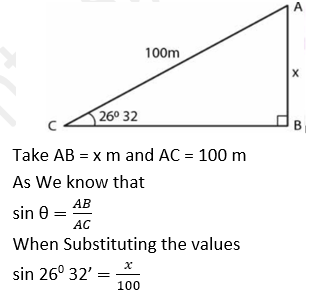So we will get
0.4467 =x/100
By further calculation
x = 100 × 0.4467
x = 44.67
= 44.7 m
Thereafter, the height of the kite is 44.7 m.

9. An electric pole is 10 m high. A steel wire tied to the top of the pole is affixed at a point on the ground to keep the pole upright. If the wire makes an angle of 450 with the horizontal through the foot of the pole, find out the length of the wire
Solution:
Let us Assume,  AB as the pole and AC as the wire which makes an angle of 450 with the ground. Height of the pole AB = 10 m
Consider x m as the length of wire AC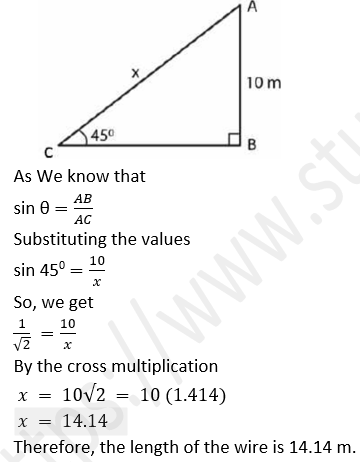10. A bridge across a river makes an angle of 45° with the river bank. If the length of the bridge across the river is 200 meters ,Find out  the breadth of the river.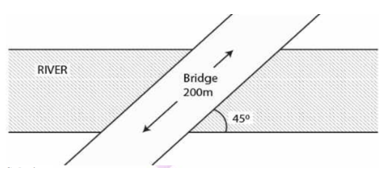Solution:
Let us Assume  AB as the width of river = x m
Length of bridge AC = 200 m
Angle with the river bank = 450
As We know that
sin θ = AB/AC
Substituting the values11. A vertical tower is 20 m high. A man standing at some distance from the tower knows that the cosine of the angle of elevation of the top of the tower is 0.53. Find out How far is he standing from the foot of the tower?
Solution:
Let us assume,  AB as the tower
Take a man C stands at a distance x m from the foot of the tower
cos θ = 0.53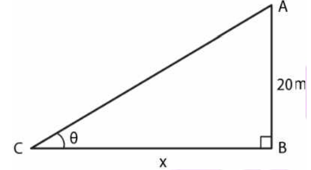As We know that
Height of the tower AB = 20 m
cos θ = 0.53
So we get θ = 58°
Let us take
tan θ = AB/CB
Substituting the values
tan 58° = 20/x
So we get
1.6003 = 20/x
By cross multiplication
x = 20/1.6003
x = 12.49
= 12.5 m
Therefore , the height of the tower is 12.5 m.

12. The upper part of a tree broken by wind falls to the ground without being detached. The top of the broken part touches the ground at an angle of 380 30’ at a point 6 m from the foot of the tree. Find out
(i) the height at which the tree is broken.
(ii) the original height of the tree correct to two decimal places.
Solution:
Lets Assume  TR as the total height of the tree
TP as the broken part which touches the ground at a distance of 6 m from the foot of the tree which makes an angle of 38° 30’ with the ground
Take PR = x and TR = x + y
PQ = PT = y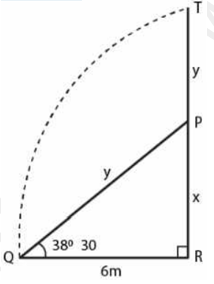In right triangle PQR
tan θ = PR/QR
Substituting the values
tan 38° 30’ = x/6
x/6 = 0.7954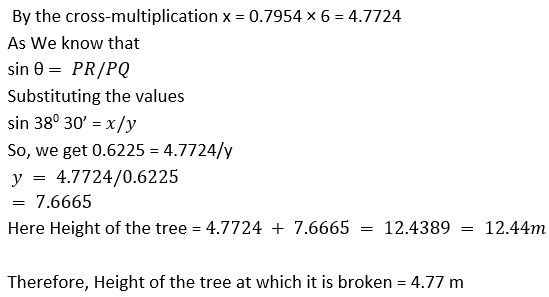13. An observer 1.5 m tall is 20.5 meters  away from a tower 22 meters high. Find out  the angle of elevation of the top of the tower from the eye of the observer.
Solution:
In the figure,
AB is the tower and CD is an observer
θ is the angle of observation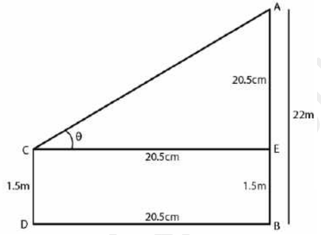It is given that
AB = 22m
CD = 1.5 m
Distance BD = 20.5 m

From the point C construct CE parallel to DB
AE = 22 – 1.5 = 20.5 m
CE = DB = 20.5 m
tan θ = AE/CE
by Substituting the values
tan θ = 20.5/20.5
= 1
tan 45° = 1
θ = 45°

14. (i) In the adjoining figure, the angle of elevation from a point P of the top of a tower QR, 50 m high is 600 and that of the tower PT from a point Q is 30° . Find out the height of the tower PT, correct to the nearest meter.
(ii) From a boat 30° meters away from a vertical cliff, the angles of elevation of the top and the foot of a vertical concrete pillar at the edge of the cliff are 55° 40’ and 54° 20’ respectively. Find out  the height of the pillar correct to the nearest meter.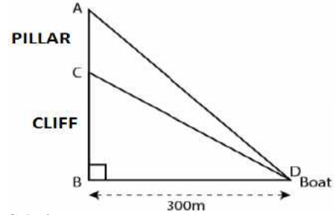Solution:-
Let us Assume  CB as the cliff and AC as the pillar
D as the boat which is 300 m away from the foot of the cliff BD = 300 m
Angle of elevation of the top and foot of the pillar are 55° 40’ and 54° 20’
Take CB = x and AC = y
In a right triangle CBD
tan θ = CB/BD
by Substituting the values
tan 54° 20’ = x/300
So we get
1.3933 = x/300
By cross multiplication
x = 300 × 1.3933
x = 417.99 m
In a right triangle ABD
tan θ = AB/BD
Substituting the values
(x + y)/ 300 = 1.4641
By cross multiplication
x + y = 1.4641 × 300 = 439.23
Substituting the value of x
y = 439.23 – 417.99 = 21.24 m = 21 m
Therefore, the height of pillar is 21 m.

15. From a point P on the ground, the angle of elevation of the top of a 10 m tall building and a helicopter hovering over the top of the building are 30° and 60° respectively. Find out the height of the helicopter above the ground.
Solution:
Lets assume  AB as the building and H as the helicopter hovering over it P is a point on the ground
Angle of elevation of the top of building and helicopter are 30° and 60°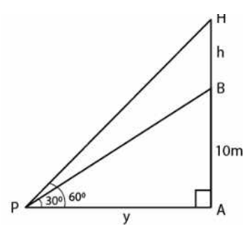As We know that
Height of the building AB = 10 m
Take PA = x m and BH = h m
In right triangle ABP
tan θ = P/B
by Substituting the values
tan 300 = AB/PA = 10/x
So we get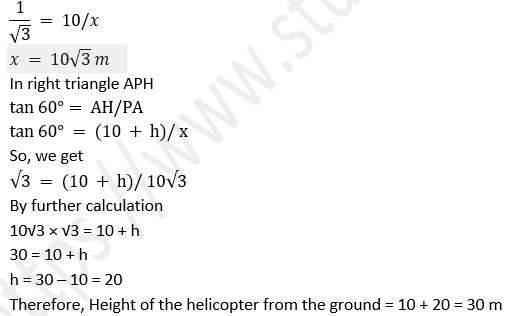16. An aeroplane when flying at a heigt of 3125 m from the ground passes vertically below another plane at an instant when the angles of elevation of the two planes from the same point on the ground are 30° and 60° respectively. Find out the distance between the two planes at the instant.
Solution:
Le us Assume the distance between two planes = h m It is given that
AD = 3125 m, ∠ACB = 60° and ∠ACD = 30°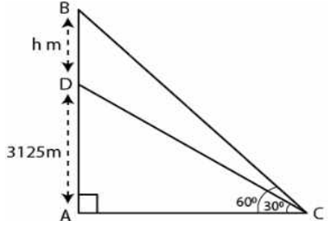In triangle ACD
Substituting the values
1/√3 = 3125/AC
AC = 3125√3  ……. (1)

In triangle ABC
tan60° = AB/AC
Substituting the values
√3 = (AD + DB)/ AC
So we get √3 = (3125 + h)/ AC
AC = (3125 + h)/ √3      …..(2)
Using both the equations
(3125 + h)/ √3 = 3125√3
By further calculation
h = (3125√3 × √3) – 3125
h = 3125 × 3 – 3125
h = 9375 – 3125
h = 6250 m

Therefore, the distance between two planes at the instant is 6250 m

17. A person standing on the bank of a river observes that the angle subtended by a tree on the opposite bank is 600 ; when he retires 20 m from the bank, he finds the angle to be 300 . Find out the height of the tree and the breadth of the river.
Solution:
Lets Assume  TR as the tree and PR as the width of the riverTake TR = X and PR = y
In right triangle TPR
tan θ = TR/PR
by Substituting the values
tan 60° = x/y
So we get
√3 = x/y
x = y √3      …… (1)
In right triangle TQR
tan 30° = TR/QR
tan 30° = x/(y + 20)
We get
1/√3 = x/(y + 20)
x = (y + 20)/ √3    …..(2)
Using both the equations
y√3 = (y + 20)/ √3
So we get
3y = y + 20
3y – y = 20
2y = 20
y = 10
Now substituting the value of y in equation (1)
x = 10 × √3 = 10 (1.732) = 17.32
Therefore , the height of the tree is 17.32 m and the width of the river is 10 m

18.The shadow of a vertical tower on a level ground increases by 10 m when the altitude of the sun changes from 45° to 30° . Find out the height of the tower, correct to two decimal places.
Solution:
In the figure
AB is the tower
BD and BC are the shadow of the tower in two situations
Let us Assume  BD = x m and AB = h mIn triangle ABD
tan 45° = h/x
So we get
1 = h/x
h = x    …..(1)
In triangle ABC
tan 30° = h/(x + 10)
So we get
1/√3 = h/(x + 10)
Using equation (1)
h√3 = h + 10
h (√3 – 1) = 10
as We know that
h = 10/(√3 – 1)
It can be written as
h = [10 (√3 + 1)]/ [(√3 – 1) (√3 + 1)]
By further calculation
h = (10√3 + 1)/ 2
So we get
h = 5 (1.73 + 1)
h = 5 × 2.73
h = 13.65 m
Therefore, the height of the tower is 13.65 m.

19. From the top of a hill, the angles of depression of two consecutive kilometer stones, due east are found to be 300 and 450 respectively. Find out the distance of two stones from the foot of the hill.
Solution:
Lets Assume A and B as the position of two consecutive kilometer stones
Here AB = 1 km = 1000 m
Take Distance BC = x m
Distance AC = (1000 + x) m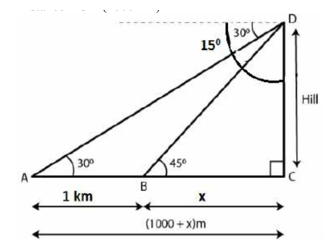In right angled triangle BCD
CD/BC = tan 45°
So we get
CD/BC = 1
CD = BC = x
In right angled triangle ACD
DC/AC = tan 30°
x/ (x + 1000) = 1/√3
By cross multiplication
√3x = x + 1000
(√3 – 1)x = 1000
x = 1000/(√3 – 1)
We can write it as
x = [1000/(√3 – 1) × (√3 + 1)/(√3 + 1)]
x = [1000 (√3 + 1)/(3 – 1)]
x = [1000 (√3 + 1)/2]
x = 500 (1.73 + 1)
So we get x = 500 × 2.73 x = 1365 m
Thereafter the distance of first stone from the foot of hill = 1365 m
Distance of the second stone from the foot of hill = 1000 + 1365 = 2365 m

20. A man observes the angles of elevation of the top of a building to be 30° . He walks towards it in a horizontal line through its base. On covering 60 m the angle of elevation changes to 60° . Find out the height of the building correct to the nearest decimal place.
Solution:
It is given that
AB is a building
CD = 60 mIn triangle ABC
tan 60° = AB/BC
√3 = AB/BC
So we get
BC = AB/√3     ….. (1)

In triangle ABD
tan 30° = AB/BD
1/√3 = AB/ (BC + 60)
By cross multiplication
BC + 60 = √3 AB
BC = √3 AB – 60
Using both the equations we get
AB/√3 = √3 AB – 60
By further calculation
AB = 3AB - 60√3
3AB – AB = 60 × 1.732
So we get
AB = (60 × 1.732)/ 2
Therefore, AB = 51.96 m

21. At a point on level ground, the angle of elevation of a vertical lower is found to be such that its tangent is 5/12. On walking 192 m towards the tower, the tangent of the angle is found to be ¾. Find out the height of the tower.
Solution:
Let us Assume  TR as the tower and P as the point on the ground such that tan θ = 5/12
tan α = ¾
PQ = 192 m
Take TR = x and QR = yIn right triangle TQR
tan α = TR/QR = x\y
So we get
3/4 = x/y
y = 4/3 x     …..(1)

In right triangle TPR
tan θ = TR/PR
Substituting the values
5/12 = x/ (y + 192)
x = (y + 192) 5/12    …… (2)
Using both the equations
x = (4/3 x + 192) 5/12
So we get
x = 5/9x + 80
x – 5/9 x = 80
4/9 x = 80
By further calculation
x = (80 × 9)/ 4 = 180
Thereafter , the height of the tower is 180 m

22. In the figure, not drawn to scale, TF is a tower. The elevation of T from A is x° where tan x = 2/5 and AF = 200 m. The elevation of T from B, where AB = 80 m, is y° . Find out :
(i) the height of the tower TF.
(ii) the angle y, correct to the nearest degree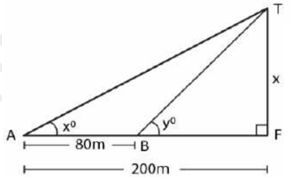Solution:-
Let us Assume  the height of the tower TF = x
It is given that
tan x = 2/5,AF = 200 m,AB = 80 m
(i) In right triangle ATF
tan x°= TF/AF
Substituting the values
2/5 = x/200
So we get
x = (2 × 200)/ 5
x = 400/5
x = 80 m
Therefore, the height of tower is 80 m.
(ii) In right triangle TBF
tan y = TF/BF
Substituting the values
tan y = 80/ (200 – 80)
tan y = 80/120
tan y = 2/3
= 0.6667
So we get
y = 30°41’
= 34°

23. From the top of a church spire 96 m high, the angles of depression of two vehicles on a road, at the same level as the base of the spire and on the same side of it are x° and y° , where tan x° = ¼ and tan y° = 1/7. Find out  the distance between the vehicles.
Solution:-
Let us Assume  CH as the height of the church
A and B are two vehicles which make an angle of depression x° and y° from C
Take AH = x and BH = yIn a right triangle CBH
tan x° = CH/AH = 96/y
by  Substituting the values
1/4 = 96/y
So we get
y = 96 × 4
= 384 m
In right triangle CAH
tan y° = CH/AH = 96/x
Substituting the values
1/7 = 96/x
So we get
x = 96 × 7
= 672 m
Here AB = x – y
AB = 672 – 384
AB = 288 m

24. In the adjoining figure, not drawn to the scale, AB is a tower and two objects C and D are located on the ground, on the same side of AB. When observed from the top A of the tower, their angles of depression are 45° and 60° . Find the distance between the two objects. If the height of the tower is 300. Give your answer to the nearest meter.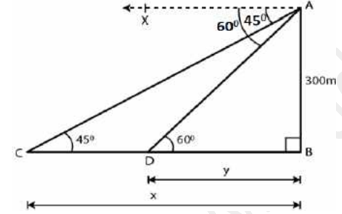Solution:
Let us assume  CB = x and DB = y
AB = 300 m
In right triangle ACD
tan θ = AB/CB
Substituting the values tan 45° = 300/x
1 = 300/x
So we get
x = 300 m
tan θ = AB/DB
Substituting the values
tan 60° = 300/y
√3 = 300/y
By further calculation
y = 300/√3
Multiply and divide by √3
y = 300/√3 × √3/√3 = 300√3/3
So we get
y = 100 × 1.732 = 173.2 m
Here CD = x – y = 300 – 173.2 = 126.8 = 127 m
Therefore, the distance between two objects is 127 m.

25. The horizontal distance between two towers is 140 m. The angle of elevation of the top of the first tower when seen from the top of the second tower is 30° . If the height of the second tower is 60 m, find out  the height of the first tower.
Solution:
Let us assume  the height of the first tower TR = x
It is given that Height of the second tower PQ = 60 m
Distance between the two towers QR = 140 mConstruct PL parallel to QR
LR = PQ = 60 m
PL = QR = 140 m
So we get
TL = (x – 60) m
In right triangle TPL
tan θ = TL/PL
Substituting the values
tan 30° = (x – 60)/ 140
So we get
1/√3 = (x – 60)/ 140
By further calculation
x – 60 = 140/√3
Multiply and divide by √3
x – 60 = 140/√3 × √3/√3 = 140√3/3
We get
x = 140√3/3 + 60
x = (140 × 1.732)/ 3 + 60
x = 80.83 + 60
x = 140.83
Therefore , the height of first tower is 140.83 m.

26. As observed from the top of a 80 m tall light house, the angles of depression of two ships on the same side of the light house in horizontal line with its base are 300 and 400 respectively. Find the distance between the two ships. Give your answer correct to the nearest meter.
Solution:
Let us assume AB as the light house and C and D as the two ships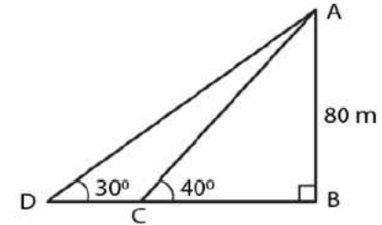tan 30°= AB/BD
Substituting the values
1/√3 = 80/BD
So we get
BD = 80√3        …… (1)
In triangle ACB
tan 40° = AB/BC
Substituting the values
0.84 = 80/BC
So we get BC = 80/0.84
= 95.25
Using equation (1)
BD = 80 √3
= 80 × 1.73
= 138.4
Here we get
DC = BD – BC DC
= 138.4 – 95.25
= 43.15
Therefore, the distance between the two ships is 43.15 m

27. The angle of elevation of a pillar from a point A on the ground is 45° and from a point B diametrically opposite to A and on the other side of the pillar is 60° . Find out the height of the pillar, given that the distance between A and B is 15 m.
Solution:.

Lets assume  CD as the pillar of x m Angles of elevation of points A and B are 45° and 60°It is given that
AB = 15 m
DB = 15 – y
Substituting the values
tan 45° = x/y
So we get
1 = x/y
x = y         …… (1)
In triangle CDB
tan 60° = x/(15 – y)
Substituting the values
√3 = x/(15 – y)
So we get
x = √3 (15 – y)    …..(2)
Using both the equations
x = √3 (15 – x)
x = 15√3 - √3x
So we get
x + √3x = 15√3
x (1 + √3) = 15√3
x = 15√3/ (1 + √3)
We can write it as
x = (15 × 1.732)/ (1 + 1.732)
x = 25.98/2.732
x = 9.51
Therefore , the height of the pillar is 9.51 m.

28. From two points A and B on the same side of a building, the angles of elevation of the top of the building are 30° and 60° respectively. If the height of the building is 10 m, find out the distance between A and B correct to two decimal places.
Solution: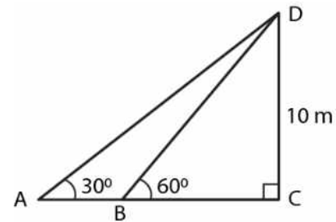In triangle DBC tan 60° = 10/BC
Substituting the values
√3 = 10/BC
BC = 10/√3

In triangle DBC
tan 30° = 10/(BC + AB)
by  Substituting the values
1/√3 = 10/[10/√3 + AB]
By further calculation
1/√3 [10/√3 + AB] = 10
So we get
AB = 10√3 – 10/√3
Taking LCM
AB = (30 – 10)/ √3
AB = 20/√3
AB = 20√3/3
So we get
AB = (20 × 1.732)/ 3
AB = 20 × 0.577
AB = 11.540 m
Therefore , the distance between A and B is 11.54 m

29. (i) The angles of depression of two ships A and B as observed from the top of a light house 60 m high are 60° and 45° respectively. If the two ships are on the opposite sides of the light house, find out the distance between the two ships. Give your answer correct to the nearest whole number.
(ii) An aeroplane at an altitude of 250 m observes the angle of depression of two boats on the opposite banks of a river to be 450 and 600 respectively. Find out the width of the river. Write the answer correct to the nearest whole number.
Solution:-
(i) Lets assume  AD as the height of the light house CD = 60 m
Take AD = x m and BD = y m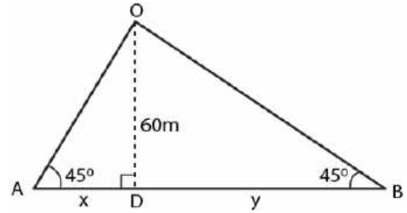In triangle ACD
Substituting the values
√3 = 60/x
So we get
x = 60/√3
Multiply and divide by √3
x = 60/√3 × √3/√3 = 60√3/3
x = 20 × 1.732
= 34.64 m
In triangle BCD
tan 45° = CD/BD
by Substituting the values
1 = 60/y
y = 60 m
Here the distance between two ships = x + y
= 34.64 + 60
= 94.64 m
= 95 m
(ii) In triangle OMA
tan 45° = OM/AM
Substituting the values
1 = 250/x
So we get
x = 250 mIn triangle OMB
tan 60° = 250/y
by Substituting the values
√3 = 250/y
So we get
y = 250/√3 = 250/1.73
y = 144.34
Here Width of the river
= x + y
Substituting the values
= 250 + 144.34
= 394.34 m

30. From a tower 126 m high, the angles of depression of two rocks which are in a horizontal line through the base of the tower are 16° and 12° 20’. Find out  the distance between the rocks if they are on
(i) the same side of the tower
(ii) the opposite sides of the tower.
Lets assume  CD as the tower of height = 126 m
A and B are the two rocks on the same line
Angles of depression are 16° and 12° 20’
Solution:Substituting the values
tan 16° = 126/x 0.2867
= 126/x
So we get
x = 126/0.2867
x = 439.48
In right triangle CBD
tan 12° 20’ = 126/y
So we get
0.2186 = 126/y
y = 126/0.2186
= 576.40
(i) In the first case
On the same side of the tower
AB = y – x
Substituting the values
AB = 576.40 – 439.48
AB = 136.92 m
(ii) In the second case
On the opposite side of the tower
AB = y + x
Substituting the values
AB = 576.40 + 439.48
Therefore ,  AB = 1015.88m

31. A man 1.8 m high stands at a distance of 3.6 m from a lamp post and casts a shadow of 5.4 m on the ground. Find out the height of the lamp post.
Solution:
Let us assume
AB as the lamp post
CD is the height of man
BD is the distance of man from the foot of the lamp
FD is the shadow of man Construct
CE parallel to DB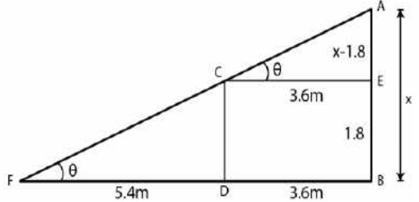Take AB = x and CD = 1.8 m
EB = CD = 1.8 m
AE = x – 1.8
FD = 5.4 m
In right triangle ACE
tan θ = AE/CE
Substituting the values
tan θ = (x – 1.8)/ 3.6 …… (1)
In right triangle CFD
tan θ = CD/FD
Substituting the values
tan θ = 1.8/5.4 = 1/3 ….. (2)
Using both the equations
(x – 1.8)/ 3.6 = 1/3
So we will get
3x – 5.4 = 3.6
3x = 3.6 + 5.4
= 9.0
By division
x = 9/3
= 3.0
Therefore,  the height of lamp post is 3 m.

32. The angles of depression of the top and the bottom of an 8 m tall building from the top of a multistoreyed building are 300 and 450 respectively. Find out the height of tire multi-storeyed building and the distance between the two buildings, correct to two decimal places.
Solution:
Lets assume  AB as the height and CD as the building
The angles of depression from A to C and D are 30° and 45°
∠ACE = 30° and ∠ADB = 45°
CD = 8 m
Take AB = h and BD = x
From the point C Construct CE parallel to DB
CE = DB = x
EB = CD = 8 m
AR = AB – EB
= h – 8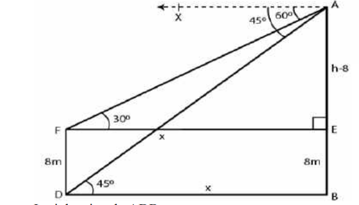tan θ = AB/DB
by Substituting the values
tan 45° = h/x
So we get
1 = h/x
x = h
In right triangle ACE
tan 30° = AE/CE
Substituting the values
1/√3 = (h – 8)/h
By further calculation
h = √3h - 8√3
So we get
√3h – h = 8√3
h (√3 – 1) = 8√3
h = 8√3/(√3 – 1)
Multiply and divide by √3 + 1
h  = 8√3/ (√3 – 1) × (√3 + 1)/(√3 + 1)
h = 8 (3 + √3)/(3 – 1)
Here
h = 8 (3 + 1.732)/2
h = 4 × 4.732
h = 18.928
h = 18.93 m
x = h = 18.93 m

Therefore
Height of multi-storeyed building = 18.93 m
Distance between the two buildings = 18.93 m

33. A pole of height 5 m is fixed on the top of a tower. The angle of elevation of the top of the pole as observed from a point A on the ground is 60° and the angle of depression of the point A from the top of the tower is 45° . Find out  the height of the tower. (Take √3 = 1.732)
Solution:
Lets assume  QR as the tower
PQ as the pole on it
Angle of elevation from P to a point A is ∠PAR = 60°
Angle of depression from Q to A = 45°
Here
∠QAR = 45°  which is the alternate angle
PQ = 5 m
Take QR = h m
PQ = 5 + hIn right triangle QAR
tan θ = QR/AR
by Substituting the values
tan 45° = h/AR
So we get
1 = h/AR
AR = h
In right triangle PAR
tan 60° = PR/AR
by Substituting the values
√3 = (5 + h)/ h
So we get
√3h = 5 + h
h (√3 – 1) = 5
h (1.732 – 1)
= 5
By further calculation
0.732 h = 5
h = 5/0.732
= 5000/732
h = 6.83
Therefore , the height of the tower is 6.83 m.

34. A vertical pole and a vertical tower are on the same level ground. From the top of the pole the angle of elevation of the top of the tower is 60° and the angle of depression of the foot of the tower is 30° . Find out the height of the tower if the height of the pole is 20 m.
Solution:
Therefore , TR as the tower
PL as the pole on the same level
Ground PL = 20 m
From the point P construct PQ parallel to LR
∠TPQ = 600 and ∠QPR = 300
Here
∠PRL = ∠QPR = 30° which are the alternate angles
Take LR = x and TR = h
TQ = TR – QR = (h – 20) mIn right triangle PRL
tan θ = PL/LR
by  Substituting the values
tan 30° = 20/x
So we get
1/√3 = 20/x
x = 20√3 m
In right triangle PQT
tan 60° = TQ/PQ
Substituting the values
√3 = (h – 20)/ x
√3 = (h – 20)/ 20√3
By cross multiplication
20√3 × √3 = h – 20
20 × 3 = h – 20
h = 60 + 20
= 80 m
Therefore , the height of the tower is 80 m.

35. From the top of a building 20 m high, the angle of elevation of the top of a monument is 450 and the angle of depression of its foot is 15° . Find out  the height of the monument.
Solution:
Lets Assume AB as the building where AB = 20 m
CD as the monument where CD = x m
Take the distance between the building and the monument as yIn right triangle BCD
tan θ = CD/BD
Substituting the values
tan 45° = x/y
1 = x/y
x = y       …… (1)
In right triangle ABD
tan 15° = AB/BD
= 20/x
Substituting the values
0.2679 = 20/x
So we get
x = 20/0.2679
= 74.65 m
Therefore, the height of the monument is 74.65 m

36. The angle of elevation of the top of an unfinished tower at a point distant 120 m from its base is 45° .find out How much higher must the tower be raised so that its angle of elevation at the same point may be 60° ?
Solution:
Lets assume  AB as the unfinished tower where AB = 120 m
Angle of elevation = 45°
Take x be higher raised so that the angle of elevation becomes 60°
BC = yIn right triangle ABC
tan θ = AB/CB
Substituting the values
tan 45° = AB/CB = 120/y
So we get
1 = 120/y
y = 120 m
In right triangle DBC
tan 60° = DB/CB
Substituting the values
√3 = (120 + x)/ 120
120√3 = 120 + x
x = 120√3 – 120
x = 120 (√3 – 1)
So we get
x = 120 (1.732 – 1)
x = 120 (0.732)
x = 87.84 m
Therefore , the tower should be raised at 87.84 m

37. In the adjoining figure, the shadow of a vertical tower on the level ground increases by 10 m, when the altitude of the sun changes from 45° to 30° . Find out the height of the tower and give your answer, correct to 1/10 of a meter.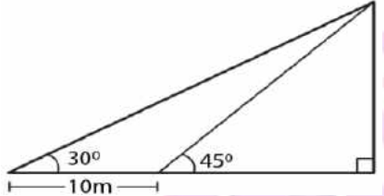Solution:
Let us Assume TR as the tower where TR = h
BR = x
AB = 10 m
Angles of elevation from the top of the tower at A and B are 30° and 45°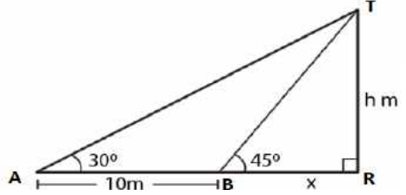In right triangle TAR
tan θ = TR/AR
Substituting the values
tan 30°= h/ (10 + x)
So we get
1/√3 = h/ (10 + x)
h = (10 + x)/ √3 …. (1)

In triangle TBR
tan 45° = TR/BR = h/x
So we get
1 = h/x
x = h   …..(2)

Using both the equations
h = (10 + h)/ √3
√3h = 10 + h
By further calculation
√3h – h = 10
(1.732 – 1) h = 10
0.732 h = 10
h = 10/0.732
= 13.66
Therefore, the height of the tower is 13.7 m.

38. An aircraft is flying at a constant height with a speed of 360 km/h. From a point on the ground, the angle of elevation of the aircraft at an instant was observed to be 45° . After 20 seconds, the angle of elevation was observed to be 30° . Find out  the height at which the aircraft flying. (use √3 = 1.732)
Solution:
As we have  given that
Speed of aircraft = 360 km/h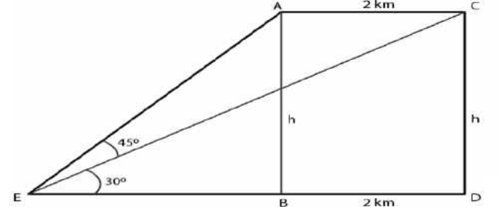Distance covered by the aircraft in 20 seconds
= (360 × 20)/ (60 × 60) = 2 km
Consider E as the fixed point on the ground CD as the position of AB in height of aircraft

Take AB = CD = h km
In right triangle ARB  tan θ = AB/ EB
Substituting the values
tan 45° = h/EB
1 = h/EB
EB = h
Here
ED = EB + BD = h + 2 km

In right triangle CED
tan 30°= CD/ED
Substituting the values
1/√3 = h/ (h + 2)
√3h = h + 2
1.732h – h = 2
0.732h = 2

As We know that 2km = 2000 m
h = 2000/0.732
h = (2000 × 1000)/732
= 2732 m

Chapter Test

1. The angle of elevation of the top of a tower from a point A (on the ground) is 30° . On walking 50 m towards the tower, the angle of elevation is found to be 60° . Find out
(i) the height of the tower (correct to one decimal place).
(ii) the distance of the tower from A.
Solution:
Let us Assume TR as the tower and A as the point on the ground
Angle of elevation of the top of tower = 30°
AB = 50 m
Angle of elevation from B = 60°
Take TR = h and AR = x
BR = x – 50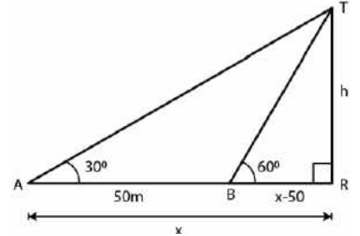In right triangle ATR
tan θ = TR/AR
by Substituting the values
tan 30°= h/x
So we get
1/√3 = h/x
x = √3h …….(1)
In right triangle BTR
tan θ = TR/BR
Substituting the values
tan 60° = h/ (x – 50)
So we get
√3 = h/ (x – 50)
h = √3 (x – 50) …… (2)
Using both the equations
h = √3 (√3h – 50)
By further calculation
h = 3h - 50√3
2h = 50√3
h = 25 √3
So we will  get
h = 25 × 1.732
= 43.3
Now substituting the values of h in equation (1)
x = √3 × 25√3
x = 25 × 3
x = 75

Therefore
Height of the tower = 43.3 m
Distance of A from the foot of the tower = 75 m

2. An aeroplane 3000 m high passes vertically above another aeroplane at an instant when the angles of elevation of the two aeroplanes from the same point on the ground are 60° and 45° respectively. Find out the vertical distance between the two planes.
Solution:
Let us assume  A and B as the two aeroplanes
P is a point on the ground such that 600 and 450 are the angles of elevations from A and B
AC = 3000 m
Take AC = 3000 m
BC = 3000 – x
PC = yIn right triangle APC
tan θ = AC/PC
Substituting the values
tan 60° = 3000/y
So we get
√3 = 3000/y
y = 3000/√3          …..(1)
In right triangle BPC
tan θ = BC/PC
Substituting the values
tan 45° = (3000 – x)/y
So we get
1 = (3000 – x)/ y
y = 3000 – x
Using equation (1)
3000/√3 = 3000 – x
By further calculation
x = 3000 – 3000/√3

Multiply and divide by √3
x = 3000 – (3000 × √3)/(√3× √3)
So we get
x = 3000 – 1000 (1.732)
x = 3000 – 1732
x = 1268
Therefore , the distance between the two planes is 1268 m.

3. A 7 m long flagstaff is fixed on the top of a tower. From a point on the ground, the angles of elevation of the top and bottom of the flagstaff are 45° and 36° respectively. Find out the height of the tower correct to one place of decimal.
Solution:
Lets assume  TR as the tower and PT as the flag on it
PT = 7 m
Take TR = h and AR = x
Angles of elevation from P and T are 45° and 36°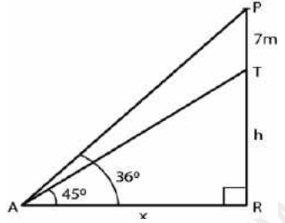In right triangle PAR
tan θ = PR/AR
Substituting the values
tan 45° = (7 + h)/ x
So we get
1 = (7 + h)/ x
x = 7 + h ….(1)

In right triangle TAR
tan θ = TR/AR
Substituting the values
tan 36° = h/x
So we get
0.7265 = h/x
h = x (0.7265) …… (2)

Using both the equations
h = (7 + h) (0.7265)
By further calculation
h = 7 × 0.7265 + 0.7265h
h – 0.7265h = 7 × 0.7265
So we get
0.2735h = 7 × 0.7265
By division
h = (7 × 0.7265)/0.2735
as We can write it as
h = (7 × 7265)/2735
h = 18.59
= 18.6 m
Therefore , the height of the tower is 18.6 m.

4. A boy 1.6 m tall is 20 m away from a tower and observes that the angle of elevation of the top of the tower is 60° . Find out the height of the tower.
Solution:

Lets assume AB as the boy and TR as the tower
AB = 1.6 m

Take TR = h
From the point A construct AE parallel to BR
ER = AB = 1.6 m
TE = h – 1.6
AE = BR = 20 mIn right triangle TAE
tan θ = TE/AE
Substituting the values
tan 60° = (h – 1.6)/20
So we get
√3 = (h – 1.6)/20
h – 1.6 = 20√3
h = 20√3 + 1.6
h = 20 (1.732) + 1.6
By further calculation
h = 34.640 + 1.6
h = 36.24
Therefore , the height of the tower is 36.24 m.

5. A boy 1.54 m tall can just see the sun over a wall 3.64 m high which is 2.1 m away from him. Find out the angle of elevation of the sun.
Solution:
Lets assume  AB as the boy and CD as the wall which is at a distance of 2.1 m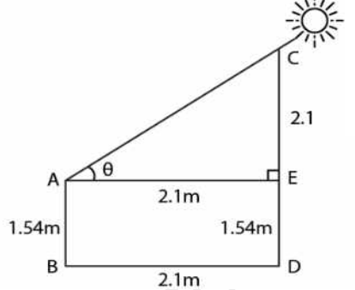AB = 1.54 m
CD = 3.64 m
BD = 2.1 m
Construct AE parallel to BD
ED = 1.54 m
CE = 3.64 – 1.54 = 2.1 m
AE = BD = 2.1 m

In right triangle CAE
tan θ = CE/AE
So we get
tan θ = 2.1/2.1
tan θ = 21/21
tan θ = 1
As We know that
tan 45° = 1
We get θ = 45°

Therefore , the angle of elevation of the sun is 45°

6. In the adjoining figure, the angle of elevation of the top P of a vertical tower from a point X is 60° ; at a point Y, 40 m vertically above X, the angle of elevation is 45° . Find out
(i) the height of the tower PQ
(ii) the distance XQ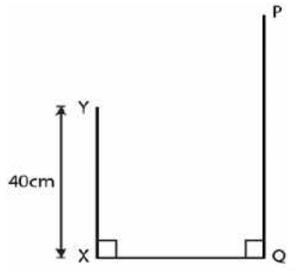Solution:

Lets assume  PQ as the tower = h
XQ = YR = y
XY = 40 m
PR = h – 40In right triangle PXQ
tan θ = PQ/XQ
Substituting the values
tan 600 = h/y
So we get
√3 = h/y
y = h/√3 …..(1)

In right triangle PYR
tan θ = PR/YR
by Substituting the values
tan 45° = (h – 40)/y
So we get
1 = (h – 40)/y
y = h – 40 …… (2)

Using both the equations
h – 40 = h/√3
By further calculation
√3h - 40√3 = h
√3h – h = 40√3

So we get
(1.732 – 1)h = 40 (1.732)
732h = 69.280
By division
h = 69.280/0.732
= 69280/732
= 94.64

Therefore Height of the tower = 94.64 m
= 95 m
Distance XQ = h – y
= 95 – 40
= 55 m

7. An aeroplane is flying horizontally 1 km above the ground is observed at an elevation of 60° . After 10 seconds, its elevation is observed to be 30° . Find out the speed of the aeroplane in km/hr.
Solution:
Lets assume  A and D as the two positions of the aeroplane
AB is the height and P is the point
AB = 1 km

Take AD = x and PB = y
Angles of elevation from A and D at the point P are 60° and 30° Construct DC perpendicular to PB
DC = AB = 1 km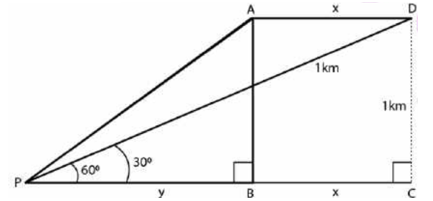In right triangle APB
tan θ = AB/PB
Substituting the values
tan 60° = 1/y
So we get
√3 = 1/y
y = 1/√3 …..(1)
In right triangle DPC
tan θ = DC/PC
Substituting the values
tan 30° = 1/ (x + y)
So we get
1/√3 = 1/ (x + y)
x + y = √3 …..(2)

Using both the equations
x + 1/√3 = √3
By further calculation
x = √3 – 1/√3
x = (3 – 1)/ √3
x = 2/√3
Multiply and divide by √3
x = (2 × √3)/ (√3 × √3)
So we get
x = (2 × 1.732)/3
x = 3.464/3 km
This distance is covered in 10 seconds

Speed of aeroplane (in km/hr) = 3.464/3 × (60 × 60)/10
By further calculation
= 3464/(3 × 1000) × 3600/10
So we get
= (3646 × 36)/300
= (3464 × 12)/100
= 41568/100
= 415.68 km/hr

8. A man on the deck of a ship is 16 m above the water level. He observes that the angle of elevation of the top of a cliff is 45° and the angle of depression of the base is 30° . find out  the distance of the cliff from the ship and the height of the cliff.
Solution:
Lets assume  A as the man on the deck of a ship B and CE is the cliff
AB = 16 m
Angle of elevation from the top of the cliff = 45°
Angle of depression at the base of the cliff = 30°
Take CE = h,AD = x
CD = h – 16Substituting the values
tan 45° = (h – 16)/x
So we get
1 = (h – 16)/x
x = h – 16 …….(1)
Substituting the values
tan 30° = 16/x
So we get
1/√3 = 16/x
x = 16√3 …… (2)

Using both the equations
h – 16 = 16 √3
h = 16√3 + 16
Taking out the common terms
h = 16 (1.732 + 1)
h = 16 (2.732)
h = 43.712
= 43.71 m

By  Substituting the value in equation (1)
x = h – 16
x = 43.71 – 16
x = 27.71
Here Distance of cliff = 27.71 m
Height of cliff = 43.71 m

9. There is a small island in between a river 100 meters wide. A tall tree stands on the island. P and Q are points directly opposite to each other on the two banks and in the line with the tree. If the angles of elevation of the top of the tree from P and Q are 30° and 45° respectively, find out  the height of the tree.
Solution:
Width of the river PQ = 100 m
B is the island and AB is the tree on it
Angles of elevation from A to P and Q are 30° and 45°
Let us assume  AB = h
PB = x
BQ = 100 – x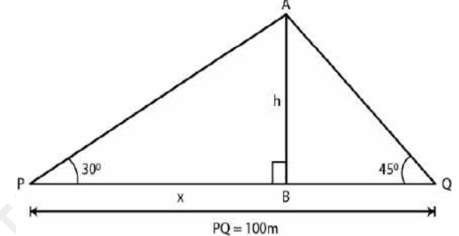In right triangle APB
tan θ = AB/PB
Substituting the values
tan 30° = h/x
So we get
1/√3 = h/x
x = √3h ….. (1)

In right triangle ABQ
tan θ = AB/BQ
Substituting the values
tan 45° = h/(100 – x)
So we get
1 = h/(100 – x)
h= 100 – x ….. (2)

Using both the equations
h = 100 - √3h
By further calculation
h + √3h = 100
So we get
(1 + 1.732) h = 100
h = 100/2.732
Multiply and divide by 1000
h = (100 × 1000)/2732
h = 100000/2732
h = 36.6

Therefore , the height of the tree is 36.6 m.

10. A man standing on the deck of the ship which is 20 m above the sea-level, observes the angle of elevation of a bird as 30° and the angle of depression of its reflection in the sea as 60° . Find out the height of the bird.
Solution:
Lets assume  P as the man standing on the deck of the ship which is 20 m above the sea level and B is the bird Angle of elevation of the bird from P = 30°
Angle of depression from P to the shadow of the bird in the sea = 60°Take BC = h
PQ = 20 m = CA
AR = (h + 20)m
CE = h + 20 + 20 = h + 40 m
PC = CA = x

In right triangle PCB
tan 30° = BC/PC
Substituting the values
1/√3 = h/x
So we get
x = √3h m ……. (1)

In right triangle PCR
tan 60° = CR/PC
Substituting the values
√3 = (h + 40)/x
Using equation (1)
(h + 40)/ √3h = √3
h + 40 = √3 × √3h = 3h
By further calculation
3h – h = 40
2h = 40
h = 40/2
= 20
Therefore From the sea level the height of the bird = 20 + h = 20 + 20 = 40 m